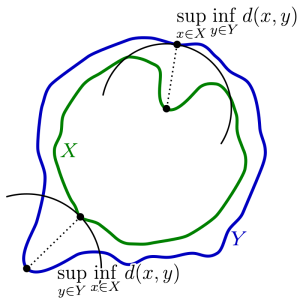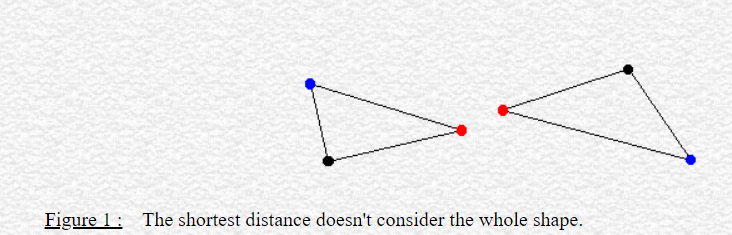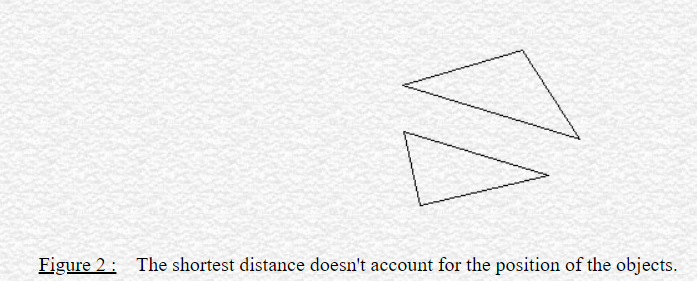Categories

# Finding the Lower Bounds of the Hausdorff Distance

Motivation: Objects are geometrically complex. There are different ways to compare objects to each other via a range of geometry processing techniques and geometric properties. Distance is often a common metric of comparison. But what type of distance should we use, which distances are favorable, and why? These are important questions…

Author: Bryce Van Ross

Currently, we’re finishing the first half of the 2-week Robust computation of the Hausdorff distance between triangle meshes project. This research is lead by mentor Dr. Leonardo Sacht, TA Erik Amézquita, and in my immediate team, I work with fellow students Deniz Ozbay and Talant Talipov. The below is a brief summary of  what’s happened so far:

A mathematical visualization of the Hausdorff distance of two meshesApplications: Primarily computer vision, computer graphics, digital fabrication, 3D-printing, and modeling. For example, in computer vision, it is often desirable to identify a best-candidate target relative to some initial template. In reference to the set of points within the template, the Hausdorff distance can be computed for  each potential target. The target with the minimum Hausdorff distance would qualify as being the best fit, ideally being a close approximation to the template object.

Motivation: Objects are geometrically complex. There are different ways to compare objects to each other via a range of geometry processing techniques and geometric properties. Distance is often a common metric of comparison. But what type of distance should we use, which distances are favorable, and why? These are important questions.

Pitfalls of using other types of distance for triangular meshesFor our research, we focus on computing the Hausdorff distance $$h$$. “Hausdorff” may seem familiar to you if you know topology. There, a (topological) space is considered Hausdorff if any two elements can be separated into disjoint (open) sets. The key idea here is the separation property with respect to points.

In geometry processing, this idea is extended (in some sense) to the separation of triangle meshes. The Hausdorff distance $$h$$ is fundamentally a maximum distance among desirable distances between 2 meshes. These desirable distances are minimum distances of all possible vectors resulting from points taken from the first mesh to the second mesh. But why is $$h$$ significant? If $$h$$ converges to zero (the smallest possible distance), then this implies that our meshes, and therefore the objects themselves, are very similar. This, like most things in math, implies within some epsilon, representing marginal change such as a slight deformation, rotation, translation, compression, or stretch. If $$h$$ is large, then this implies that the two objects are dissimilar. Intuitively, this is due to a lack of ideal correspondence from triangle to triangle. In short, $$h$$ serves as a means of computing the similarity between digital objects in terms of maximally separating the meshes’  points according to their minimum distances.

Tasks: To compute $$h$$, we find the maximizer, the point (in the first mesh) corresponding to the computation of $$h$$. This point is found via an algorithmic process called the branch and bound technique. Sparing the details, the result of applying this technique will provide a (very small) region where the minimizer is claimed to exist, after a series of triangle subdivisions and deletions. There are different ways to implement this technique. Our collective goal this week was to achieve accurate $$h$$ given any two meshes. Once this is ensured, Week 2 would focus on making our code more robust (efficient/fast). This work can be simplified into three primary tasks: encoding the lower bound and upper bound of possible values of $$h$$, and the subdivision method. My work focused on the lower bound, for which the other two functions are dependent.

Accomplishments: By Day 2, we started with writing pseudocode for the lower bound, using only the vertices of the first mesh and computing their distances with respect to vertices of the second mesh. This was computed per face, and the minimum was found. Although this method was correct, it didn’t account for either the edge or interior cases of a given triangle. Looping through an edge would be immediately doable, but the interior case would be more challenging. Thankfully, Dr. Sacht had us search through gptoolbox for such functionality that would account for all three cases. Once finding this function, we were able to reduce my code to two lines! The irony is although this was valid, the referenced function was basically an empty shell. The function was actually calling a C++ function that would have to compiled and linked against other libraries and due to our time constraints we decided it was best to address this issue in a future time. Ultimately, we ended up having to write from scratch once more. In searching for similar point-triangle distance algorithms, we initially found an approach using normal vectors, which would offer projective power of the former mesh vertices relative to the latter mesh. The computations were erroneous and misrepresentative. Since then, our team has been using a combinatorial plane-vector approach to find the lower bounds per vertex.

Hopefully we’ll finish soon… we’re excited for the next steps!

## One reply on “Finding the Lower Bounds of the Hausdorff Distance”

[…] We define the lower bound as the minimum of the distances from all the vertices of mesh A to mesh B. Firstly, we choose the vertex P on mesh A. Secondly, we compute the distances from point P  to all the faces of mesh B. The actual distance from point P to mesh B is the minimum of the distances that were calculated the step before. For more theoretical details you should check this blog post: http://summergeometry.org/sgi2021/finding-the-lower-bounds-of-the-hausdorff-distance/ […]